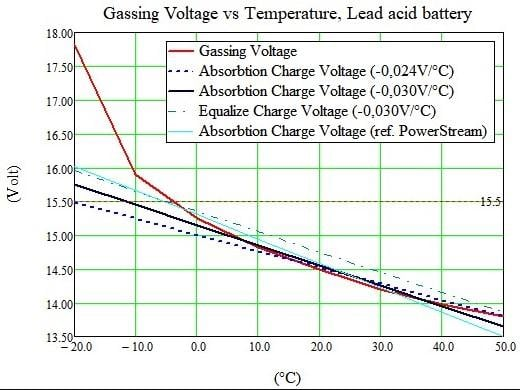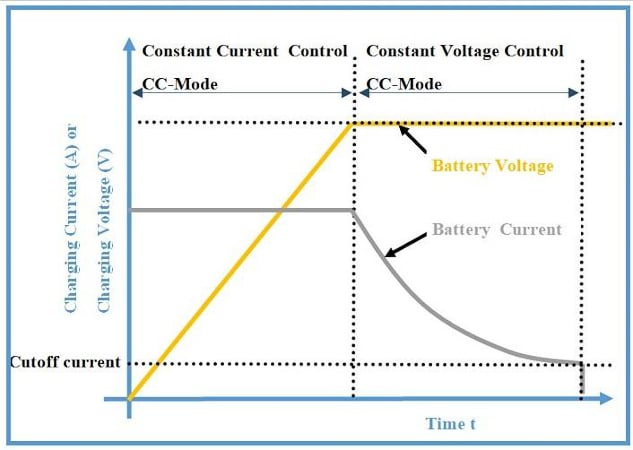Technical Article

# Gassing Voltage in Battery Charging: Mitigation Strategies

April 13, 2023 by Bob Odhiambo

## This article discusses what gassing voltage is, how it affects battery health, and methods of preventing and mitigating it.

Batteries power electronic devices that are an integral part of our daily lives, from small electrical gadgets to electric cars. To properly maintain the functionality of the batteries, it is vital to understand the complexities of battery charging, including the phenomenon of gassing voltage.

### Gassing Voltage in Battery Chargers

Gassing voltage occurs during battery charging due to water electrolysis. The internal pressure of the battery causes the release of hydrogen gas. The voltage produced at the point of the internal pressure is the gassing voltage. A hissing sound often accompanies the production of hydrogen gas. Temperature, battery type, and state of charge affect gassing voltage.

### Calculating Gassing Voltage

Calculating gassing voltage involves factors such as charging rate, temperature, and battery chemistry. There is a variance in the specific formula used in calculating the gassing voltage depending on the mathematical model being used and the battery's chemistry.

In the example of lead-acid batteries, gassing voltage is estimated as:

VG = 1.78+(T × 0.007)+(C/4.4)

Where:

VG = Gassing voltage per cell (Volts)

T = Temperature (Degrees Celsius)

C = Capacity of the battery (Ah)

The above equation describes the relationship between the discharge rate and the capacity of the battery. It is based on the Peukert equation.

Below are some formulas to evaluate gassing voltage.

• Peukert’s law: This is an empirical formula relating to the discharge rate and battery capacity. The law states an increase in discharge current with a decrease in battery capacity. The relationship is shown in the formula:

I×t=Ck

Where:

I = Discharge current

t = time of discharge

k = Peukert exponent

The Peukert exponent refers to the measure of the efficiency of delivering a battery’s rated capacity at distinct discharge rates. Depending on the battery construction and chemistry, this exponent ranges from 1.1 to 1.5 in batteries like lead-acid. When the Peukert’s exponent is low, the battery delivers more capacity at higher discharge rates than the higher Peukert’s exponent, making the battery less efficient at higher discharge rates.

Peukert’s law is vital in the selection and sizing of batteries and can be used to evaluate the gassing voltage of lead-acid batteries. This is because gassing voltage depends on the battery capacity and the discharge rate. Understanding the law can help prevent damage to the battery due to excess gassing during charging.

• Nernst equation: This is an electrochemical equation relating the electrode potential and the concentration of reactants in an electrochemical cell involved in the cell reaction. The Nernst equation is expressed by:

E = E0 – (RT/Nf)InQ

Where:

E = Electrode potential

E0 = Standard electrode potential

T = Temperature

n = Number of electrons transferred in the chemical reaction

Q = Reaction quotient

The reaction quotient Q is the ratio of the product’s concentration to the concentration of the reactants with each concentration in the balanced equation raised to the power of its stoichiometric coefficient.

The Nernst equation can be used to evaluate the potential of an electrode of a half-cell in a given concentration of reactants. The equation can be used to calculate the gassing voltage based on the chemical reaction in the battery.

Battery capacity calculation: The capacity C of the battery can be calculated by multiplying the time taken t to discharge the battery with the current I flowing through the battery. The formula for calculating the battery's capacity is as follows:

C = I × t

Overall, calculating gassing voltage involves many intricate formulas specific to the battery type being used. Evaluating and monitoring battery behavior during charging is vital to accurately resolve any excess gassing voltage for safe and efficient battery operation.

### Implications of Gassing Voltage

Depending on the particular electrochemical system, gassing voltage can have either positive or negative impacts on the system.

One benefit of gassing voltage in electrochemical systems is that it can be used in the synthesis of gasses in such systems, electroplating, and electromechanical fuel cells.in the applications, gassing voltage can control the gas production rate as it is an essential part of the reaction.

On the negative, gassing voltage can pose a challenge to some electrochemical systems such as batteries. The gas buildup in the battery can cause a loss in electrolyte and electrochemical corrosion. This corrosion can result from the buildup of gas that forms bubbles that affect the protective layer of the battery.

### Mitigation Strategies

Several mitigation strategies can be used to minimize gassing voltage during the battery charging process. They include:

#### Temperature Control

The gassing voltage in a battery increases with increased temperature. Therefore, controlling the temperature is critical to prevent the excess gassing voltage. Depending on the battery's chemistry, there is a variation in the optimum temperature of the battery. For instance, lithium-ion batteries should be charged between 0o C and 45o C, while lead acid should be charged between 25o C and 35o C. The battery's life can be reduced when it is charged outside its recommended temperature due to excess gassing. In Figure 1 below, the charging limit voltage reference for the lead-acid battery is 15.5 V.##### Figure 1. Graph showing the relationship between temperature and the gassing voltage in the lead-acid battery. Image used courtesy of Bob Odhiambo

Monitoring the battery's temperature can also ensure effective temperature control during battery charging. This can be done by using sensors to acquire the battery's temperature data and adjust the charging rate accordingly.

#### Charging Algorithm

The charging algorithm of a battery is a set of instructions or programs dictating how the charging process should occur. In the algorithm, the state of charge and voltage are monitored, and the charging voltage is adjusted to meet the demand for the battery's efficiency.

Several charging algorithms can be employed; however, the most common algorithm is the constant current/constant voltage (CV/CV) algorithm. In the CV/CV algorithm, the charging current is maintained at a constant level until a certain voltage is attained where the current now reduces to maintain a constant voltage. This algorithm allows for the battery to accept high charging current at the start of the charging process, making it faster, then gradually reducing it to avoid overcharging.##### Figure 2. Graph showing the characteristics of constant current/ constant voltage charging mode with charging time. Image used courtesy of Bob Odhiambo

Other charging algorithms include the trickle charging algorithm, in which a low charging current is used to maintain the battery’s charge level and the pulse charging algorithm, in which the charging current is delivered to the battery in pulses.

#### Charge Current Limit

This is a method that can mitigate the gassing voltage in a battery by limiting the current flowing in the battery during the charging process. This is done using a charge controller to control the charging current to the battery. The charge current limit is often set based on the chemistry of the battery and its capacity. For instance, the recommended charge current for a lead-acid battery is within 10% to 13% of the battery’s capacity rating.

The implementation of charge current limiting can necessitate the design of a simple charge controller circuit or a more complex system that uses microcontrollers to monitor and adjust the charging current according to the voltage of the battery.

### Takeaways of Gassing Voltage

Knowing the gassing voltage in the battery charging process is critical in ensuring that the functionality and life of the battery are prolonged. Gassing voltage can either have a positive impact on some applications and also a negative impact on other applications. To mitigate the impact of gassing voltage in the charging process, aspects such as temperature control, monitoring the state of charge, and limiting the charging current should be considered. It is, therefore, necessary to accurately evaluate and monitor the behavior of the battery during its charging process to resolve any excess gassing voltage.

Gassing voltage occurs as a result of the electrolysis of water in batteries.

The gas released can result in electrochemical corrosion and reduce overall battery health.

The charging rate, temperature, and chemistry of the battery are factors to consider when calculating gassing voltage.

Gassing voltage can be calculated using the following formulas:

I × t = Ck (Peukert’s Law), E = E0 – (RT/Nf)InQ (Nernst Equation), and C = I × t (Battery capacity)

Featured image used courtesy of Adobe Stock